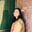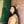Trusted answers to developer questions
Trusted Answers to Developer Questions

Related Tags

numpy
python

# What is numpy.power() in Python?Umme Ammara

Grokking Modern System Design Interview for Engineers & Managers

Ace your System Design Interview and take your career to the next level. Learn to handle the design of applications like Netflix, Quora, Facebook, Uber, and many more in a 45-min interview. Learn the RESHADED framework for architecting web-scale applications by determining requirements, constraints, and assumptions before diving into a step-by-step design process.

Python’s numpy.power() raises the first array elements to the power of second array elements. The illustration below shows this functionality:

## Syntax

numpy.power() is declared as follows:

numpy.power(x1, x2, /, out=None, *, where=True, casting='same_kind', order='K', dtype=None, subok=True[, signature, extobj]) = <ufunc 'power'>


In the syntax given above, x1 and x2 are non-optional parameters, and the rest are optional parameters.

A universal function (ufunc) is a function that operates on ndarrays in an element-by-element fashion. The power() method is a universal function.

## Parameters

The numpy.power() method takes the following compulsory parameters:

• x1 [array-like] - input array elements that act as the base.

• x2 [array-like] - input array elements that acts as the exponent. If the shapethe shape of an array is the number of elements in each dimension of x1 and x2 is different, they must be broadcastable to a common shape for representing the output.

The numpy.power() method takes the following optional parameters:

 Parameter Description out represents the location into which the output of the method is stored. where True value indicates that a universal function should be calculated at this position. casting controls the type of datacasting that should occur. The same_kind option indicates that safe casting or casting within the same kind should take place. order controls the memory layout order of the output function. The option K means reading the elements in the order they occur in memory. dtype represents the desired data type of the array. subok decides if subclasses should be made or not. If True, subclasses will be passed through.

## Return value

numpy.power() returns the bases in x1 raised to the exponents in x1.

• If x2 is negative, a ValueError is raised.

• If both x1 and x2 are scalars, the return type is scalar.

## Examples

In the example below, the bases in array arr1 are raised to the exponents in the array arr2:

import numpy as nparr1 = np.array([2, 3, 4])arr2 = np.array([2, 2, 2])print (np.power(arr1, arr2))

In the example below, the bases in array arr3 are raised to the exponents in the array arr4:

import numpy as np arr3 = np.array([[1, 2, 3], [4, 5, 6]])arr4 = np.array([[1, 2, 3], [4, 5, 6]])print (np.power(arr3, arr4))

The following example shows that the ValueError is raised when an exponent is a negative number.

import numpy as np arr5 = np.array([1, 2, 3])arr6 = np.array([-1, 3, -5])print (np.power(arr5, arr6))

RELATED TAGS

numpy
python

CONTRIBUTORUmme Ammara